Rate of reaction

• AS Chemistry introduced the qualitative aspects of rates of reaction.  These include:
 Collision theory Effect of temperature Effect of concentration Effect of pressure Activation energy Catalysis

Rate of reaction

The rate of a reaction is defined as the change in concentration of a reactant or product in a given time

 Rate = Change in concentration Units: mole dm-3 = mol dm-3 s-1 Time s
• Other units may be used that are more convenient - if a gas is produced it may be in cm3 min-1

Representing concentration

• Square brackets, [   ] is used to represent the concentration, mol dm-3 of a reactant or product.

Measuring rates

• As a reaction proceeds, the reactants get used up.  This means that the concentration decreases.
• This explains why reactions:
 SLOWS DOWN As fewer collisions take place STOPS When one reactant has been used up
• The rate can be determined by measuring the concentration of a reactant or product at regular time intervals.
• The results can then be plotted giving a concentration - time graph.
• At any point, the rate is equal to the gradient of the curve:
 y/x Concentration  /  time Gradient Rate
• The gradient of a curve is measured by drawing tangents:

A)  Measuring the decrease in concentration of a reactant: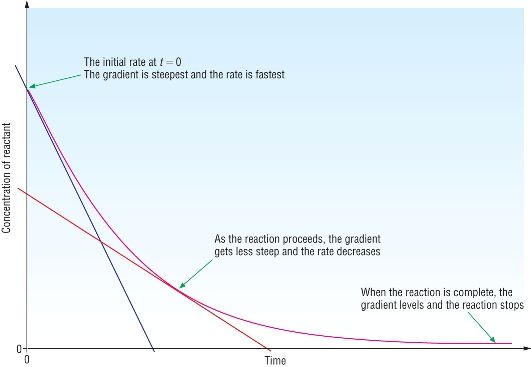• As reactants are being used up, we measure the rate of decrease in concentration.
• The gradient at t = 0 is at the start of the reaction.  We call this the initial rate.

A)  Measuring the increase in concentration of a product: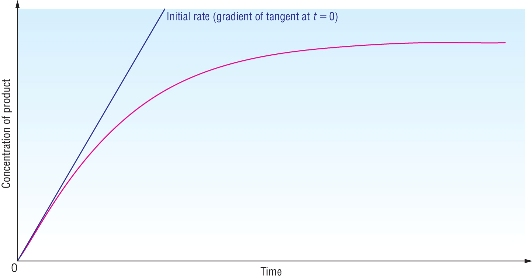• As products are being produced, we measure the rate of increase in concentration.
• The gradient at t = 0 is at the start of the reaction.  We call this the initial rate.

Qu 1 - 3   P113

Measuring reaction rates

Obtaining data for a concentration - time graph:

• Rates can be measured experimentally using the following techniques:
 Acid - Base reactions Gas production Visible changes Titrations Change in volume (gas collection) Precipitation (colorimeter) pH meter Loss in mass Colour changes (colorimeter)
• Basically we can use any method to follow a reaction that produces a measurable change.

Using a colorimeter

• Adding iron fillings to a solution of copper sulphate makes iron sulphate and copper:
 Fe(s) + CuSO4(aq) à Fe2SO4(aq) + Cu(s) Blue solution Colourless solution
• The solution gradually gets paler as the concentration of copper sulphate decreases and the concentration of iron sulphate increases.Concentration of copper sulphate solution

 1M 0.8M 0.6M 0.4M 0.2M
• A colorimeter will monitor the intensity of the colour which is proportional to the concentration of copper sulphate.

• This will produce a graph that we can use to measure the rate.

Measuring the rate from a concentration - time graph

• Sulphur dioxide decomposes producing sulphur dioxide and chlorine:

 SO2Cl2(g) à SO2(g) + Cl2(g)
• The concentration of SO2Cl2 was measured and plottedRed tangent is at t = 0 (initial rate): rate = 0.50 - 0.00 =   1.5 x 10-4 mol dm3 s-1               3300 - 0 Green tangent is at t = 3000: rate = 0.38 - 0.14 =   6.0 x 10-5 mol dm3 s-1               4000 - 0

Question 1  P 115

Orders and the rate equation

Orders:

• A reaction occurs when reactants collide with energy greater than the activation energy.
• Doubling the concentration of a reactant can have one of 3 effects on the rate of a reaction:
 1)  No effect 2)  Double the rate 3)  Quadruple the rate
• This means there is a mathematical relationship between concentration and the rate of a reaction
• The order determines the effect a change in concentration of a reactant will have on the rate:
 Rate is proportional to the concentration Rate a [A] x x is the order

The rate equation and the rate constant:

• To remove the a  we have to add a constant of proportionality.  This is called the rate constant.
• The rate then is:
 Rate is proportional to the concentration Rate = k [A] x
• To look at how the order effects the rate we'll keep the numbers simple.

• Lets make:

 k = 1 [A] = 1 Doubling the concentration of A from 1M to 2M [A] = 2

Zero order - where x = 0:

 Rate = k [A] x Rate = 1  0 Rate = 1 Now double the concentration of A Rate = 1  0 Rate = 1

Doubling the concentration has no effect on the rate

First order - where x = 1:

 Rate = k [A] x Rate = 1  1 Rate = 1 Now double the concentration of A Rate = 1  1 Rate = 2

Doubling the concentration doubles the rate wrt [A]

Second order - where x = 2:

 Rate = k [A] x Rate = 1  2 Rate = 1 Now double the concentration of A Rate = 1  2 Rate = 4

Doubling the concentration quadruples the rate wrt [A]

The rate equation and the rate constant:

• To remove the a  we have to add a constant of proportionality.  This is called the rate constant.
• The rate then is:
 Rate is proportional to the concentration Rate = k [A] x
• Remember - a reaction usually has more than one reactant:

 A + B + C à Products
• This means that the rate could depend on all 3 reactants:
 Rate a [A] 0 Rate a [B] 1 Rate a [C] 2
• Combining these 3 give:

 Rate a [A] 0 [B] 1 [C] 2
• The rate constant, k links the rate with the concentrations:
 Rate = k [A] 0 [B] 1 [C] 2
• Remember anything to the power 0 is always equal to 1, the rate equation then is:
 Rate = k [B] 1 [C] 2

Overall order:

• This is the sum of all the orders of all the reactants in the rate equation, for:
 Rate = k [B] 1 [C] 2
• Overall order is 1  +  2  3

Orders of reaction and the rate equation can only be determined experimentally.

Units of rate constants:

• The units for rate constants will vary as each reaction will have different numbers of reactants present.
• The orders will also vary.
• To work the units out rewrite the rate equation substituting the rate and concentrations with their corresponding units.
• Use algebra to cross cancel and obtain k on its own:
 Rate = k  [A] 1 Rate = k  [A] 2 Rate = k  [A]  [B] k = Rate k = Rate k = Rate [A] 1 [A] 2 [A]  [B] k = mol dm-3 s-1 k = mol dm-3 s-1 k = mol dm-3 s-1 mol dm-3 mol dm-3 mol dm-3 mol dm-3 mol dm-3 k = mol dm-3 s-1 k = mol dm-3 s-1 k = mol dm-3 s-1 mol dm-3 mol dm-3 mol dm-3 mol dm-3 mol dm-3 k = s-1 k = s-1 k = s-1 mol dm-3 mol dm-3 k = dm3 mol-1 s-1 k = dm3 mol-1 s-1

Questions 1 - 3  P117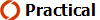Students to follow method 1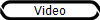and / or carry out method 2 at home

Half - lives

Concentration - time graphs:

• The concentration of a reaction is monitored then plotted against time.
• The order of the reaction wrt that reactant can be determined from the shape of the graph:

Half - life:

• This is the time taken for the concentration of a reactant to half.
• It is an important feature of concentration time graphs:

Concentration - time graph for a first order reaction:

• First order reactions half constant half lives.
• No matter what the initial concentration is, the time taken for the concentration to halve will be the same.
 2N2O(g) à 2N2(g) + O2(g)
• The reaction is first order wrt [N2O], this can be seen by the constant half - lives.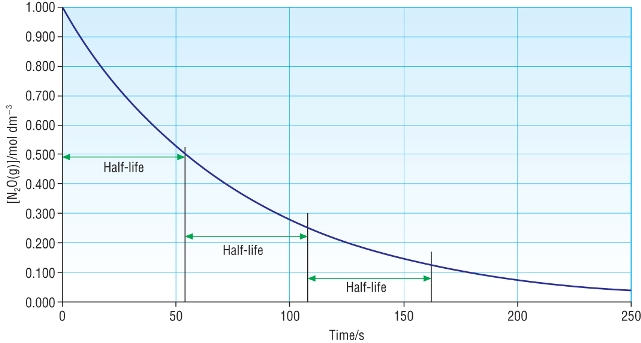Other concentration - time graphs:

• The other 2 orders have unique shapes:
 Zero order First order Second order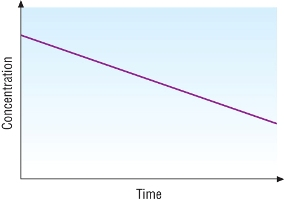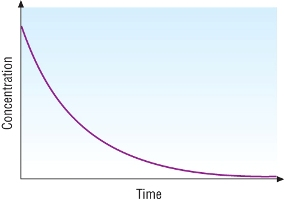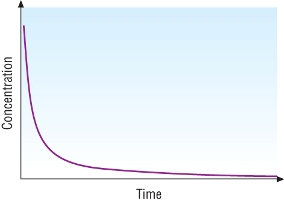Concentration decreases at a constant rate - Straight line Remember, this reactant has no effect on the rate but will get used up in the reaction. Concentration halves at equal time intervals - Constant half - lives. As the concentration halves the rate also halves. Concentration decreases rapidly - Half life increases. As the concentration halves, the rate reduces by 1/4 so half lives increase.

Qu 1 - 2  P 119

Qu 1  P159

Orders from rate - concentration graphs

• Orders can also be determined by plotting rate against concentration.
• Different orders give different shaped graphs:
 Zero order First order Second order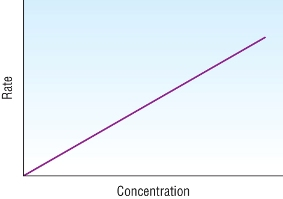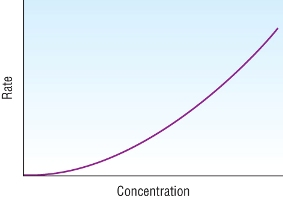Remember, this reactant has no effect on the rate. The rate continues at a steady pace.     Horizontal straight line As the concentration doubles the rate also doubles, as the concentration triples, the rate triples. The rate and concentration are in direct proportion to each other.   Proportional straight line As the concentration doubles the rate also quadruples, as the concentration triples, the rate increases by 9. The rate increases by the square of the concentration. What if rate - concentration2 was plotted? Proportional curve

Initial rates:

• Initial rates can be determined in one of 2 ways:

A)  From concentration - time graphs:

• Draw a tangent at t=0, the gradient of this tangent is the initial rate.

B)  From clock reactions:

• Clock reactions are when after a certain time a sharp change is observed:
 appearance of a precipitate disappearance of a solid a change in colour
• During these types of reactions you are measuring the rate for the initial period of the reaction.
• This means that there will not be a significant change in concentration so we can assume that the rate is jus 1/t.
• Repeating each experiment with different concentrations for one reactant then again with another reactant.
• Plot graphs for each one a determine the order.

Example - Sodium thiosulphate and hydrochloric acid:

• See the experiment:Na2S2O3(aq) + 2HCl(aq) à 2NaCl(aq) + S(s) + SO2(aq) + H2O(l)
• The experiment is repeated with different [HCl]
• And again with different [Na2S2O3]
• Graphs are plotted for each:
 Na2S2O3 HClAs the concentration doubles the rate also doubles, as the concentration triples, the rate triples. The rate and concentration are in direct proportion to each other. Proportional straight line 1st order wrt [Na2S2O3] Remember, this reactant has no effect on the rate. The rate continues at a steady pace.   Horizontal straight line zero order wrt [HCl]
 Rate = k  [Na2S2O3]1  [HCl]0
 Rate = k  [Na2S2O3]

Questions 1,2  P 121

Initial rates and the rate constant

Determination of orders by inspection:

• Sometimes it is easier to look at the raw data for different initial concentrations of reactants.
• Comparing  the rates and concentrations of the different reactants allows you to find the orders and therefore the rate equation:

Example:

• Consider the reaction:

 2NO(g) + O2(g) à 4NO2
• Some results are:
 Experiment Initial concentration [NO] (mol dm-3) Initial concentration [O2] (mol dm-3) Initial rate (mol dm-3 s-1) 1 0.00100 0.00100 1.82  x  10-6 2 0.00100 0.00300 5.46  x  10-6 3 0.00200 0.00100 7.28  x  10-6

Order for NO:

• Experiments 1 and 3:  The concentration has been doubled - The rate has quadrupled, [NO]2

• The order wrt [NO] = 2

Order for O2:

• Experiments 1 and 2  The concentration has been doubled - The rate has also doubled, [O2]1

• The order wrt [O2] = 1

Rate equation:

 Rate = k  [NO]2      [O2]1
• overall order = 3

Rate constant:

 Rate = k  [NO]2   [O2]1 Rate = k  [NO]2   [O2]1 k = Rate k = Rate [NO]2   [O2]1 [NO]2   [O2]1 k = 5.46  x  10-6 k = mol dm-3 s-1 (0.00100)2  x  (0.00300) (mol dm-3)2 mol dm-3 k = 1820 k = mol dm-3 s-1 (mol dm-3)2 mol dm-3 k = s-1 (mol dm-3)2 k = dm6 mol-2 s-1

The rate constant, k:

• The rate depends on 2 things:
 Concentration rate constant
• A fast reaction must therefore have a large rate constant if all concentrations are equal.

The effect of temperature on the rate constant

• A 10oC rise in temperature will double the rate of a reaction.
• This was explained in AS by the Boltzmann Distribution.
• The key factor is the number of collisions greater than the Activation Energy
• Look at the rate equation again:

r     =          k [A]x [B]y

• Obviously the temperature cannot change the concentrations of the reactants.
• This means that the only thing that can change is the rate constant, k.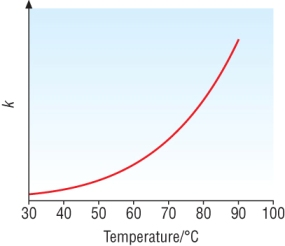• The stoichiometry for a chemical reaction gives no information for the rate equation or the rate constant.

Questions 1-2  P123

Rate - determining step

• We have already seen in the organic chemistry that there is a series of steps to an overall reaction.

• This is called a mechanism.

• Each step could occur at different rates and it usually does so.

• The overall rate of a reaction can only occur as fast as its slowest step.

• This slowest step is called the rate determining step:

Analogy - Making ham sandwiches

 Step 1 Step 2 Step 3 Get out 2 slices of bread Butter the bread add the ham between the slices and assemble the sandwich Put the sandwich in a sandwich bag
• Step 2 is the slowest step in this process so this would be the rate determining step.

• If I offered to help the students in step 1 or 3, the rate of sandwich making would not increase.

• If I offered to help the students in step 2, the rate of sandwich making would increase.

• This makes step 2 the rate determining step.

Predicting reaction mechanisms from rate equations:

• Consider the reaction:

 NO2(g) + CO(g) à NO(g) + CO2(g)
• The overall equation does not tell you anything about the rate equation or the reaction mechanism.

• The rate equation can be determined experimentally and this gives you clues to the mechanism (or at least the RDS).

• Experimental data tells us that the rate is:

 Second order wrt NO2(g) Zero order wrt CO(g)
• The rate equation then is:

r     =          k [NO2]2

• This means that NO2 must be in the rate determining step.

• As doubling the concentration has a quadrupling effect then this must mean that 2 of the NO2 molecules must be involved in the RDS.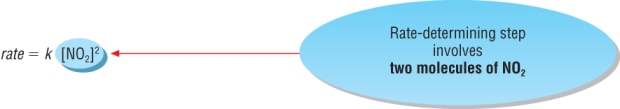• This gives us clues to the reaction mechanism:

 First step NO2 + NO2 à Slow - Rate Determining Step
• This means that the remaining steps in the mechanism must be fast steps / reactions.

• The sum of all the steps must equal the overall equation.

• CO and CO2 must be involved in the second step as they are in the overall equation

• So far we know:

 First step NO2 + NO2 à Slow - Rate Determining Step Second step + CO à + CO2 Fast Overall NO2 + CO à NO + CO2
• The sum of all of the reactants and products (including cross cancelling) must be equal to the overall equation.

• This means that the other product in the second step must be NO2 to cancel out the 2nd NO2 reactant in the first step:

 First step NO2 + NO2 à Slow - Rate Determining Step Second step + CO à NO2 + CO2 Fast Overall NO2 + CO à NO + CO2
• This means that the other reactant in the second step must be NO3 to keep the second step balanced.

• The NO3 in the second step must have been produced in the first step:

 First step NO2 + NO2 à NO3 + Slow - Rate Determining Step Second step NO3 + CO à NO2 + CO2 Fast Overall NO2 + CO à NO + CO2
• This means that the other product in first step must be NO to keep the first step balanced.

• The NO3 in the second step must have been produced in the first step:

 First step NO2 + NO2 à NO3 + NO Slow - Rate Determining Step Second step NO3 + CO à NO2 + CO2 Fast Overall NO2 + CO à NO + CO2
• The NO3 is described as an intermediate as it is produced in the first step and consumed in the second step.

• Mechanisms are an educated 'guess' for the reaction sequence and can be proved wrong.

Summary:

• Remember the rate equation cannot be determined from the stoichiometry of a reaction.
• A first order reaction with respect to 1 species suggests a unimolecular rate determining step.
• A second order reaction with respect to 1 species suggests a bimolecular rate determining step.

If a reactant appears in the rate equation, then that reactant takes part in the slow step of the reaction (RDS).  If it does not appear in the rate equation then the reactant does not participate in the slow step (RDS).

Qu 1 - 2  P 125  /  Qu 1 - 3  P 159  /  Qu 1 - 2  P161